# Electro Static: solved equations

## Introduction

In an Electro Static application the equations used for computation are:

• the corresponding Maxwell's equations for an electrical system, and
• the constitutive equations that characterize the dielectric materials

The conditions of computation of an Electro Static application are the following:

• the computation concerns the D and E fields; the B and H fields are not computed. The equations of the electric fields D, E and of the magnetic fields B, H are decoupled.
• the fields are time independent (static): d/dt = 0
• the current density is null: J = 0

## Equations and conditions

In the previously defined conditions of computation , the equations are summarized as follows:

Equations Description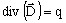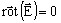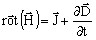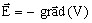E: electric field strength (in V/m)

D: electric flux density (in C/m2)

V: electric potential (in V)

q: density of electric charges (in C/m3)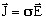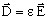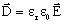εr : relative permittivity

ε0 : vacuum permittivity (in F/m)

## Solved equation

The second order equation solved by the finite element method in Flux in case of an Electro Static application is the following: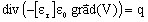where:

•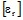is the tensor of relative permittivity of the medium
• ε0 is the permittivity of the vacuum; ε0 = 1/(36π109) (in F/m)
• V is the electric potential (in V)
• q is the density of electric charges (in C/m3)

## State variable

The state variable of the Electro Static application is the electric potential V (written Ve in Flux 3D).

The uniqueness condition of the scalar field of the electric potential V requires that the value of this potential be assigned to at least one point of the computation domain.